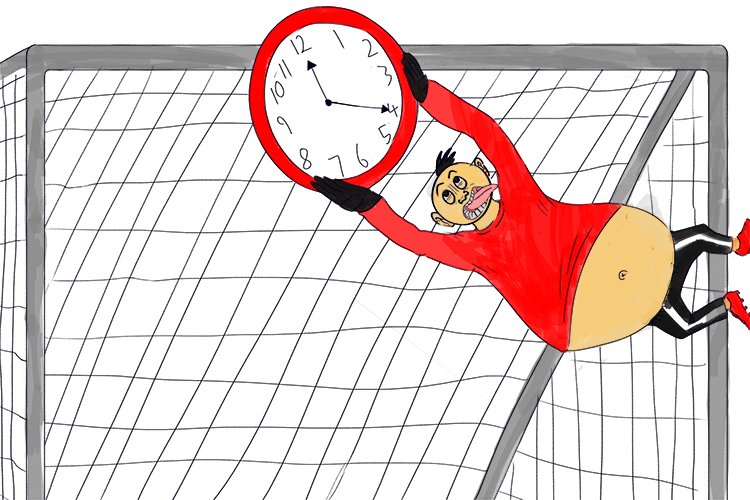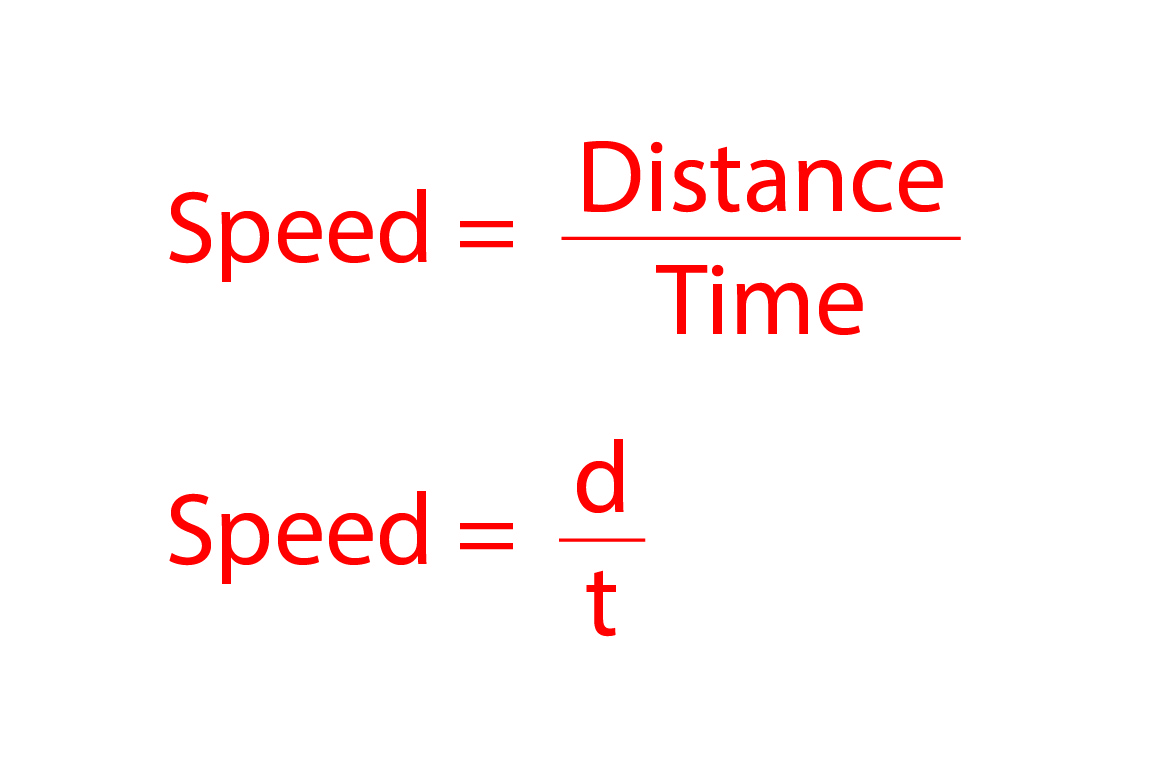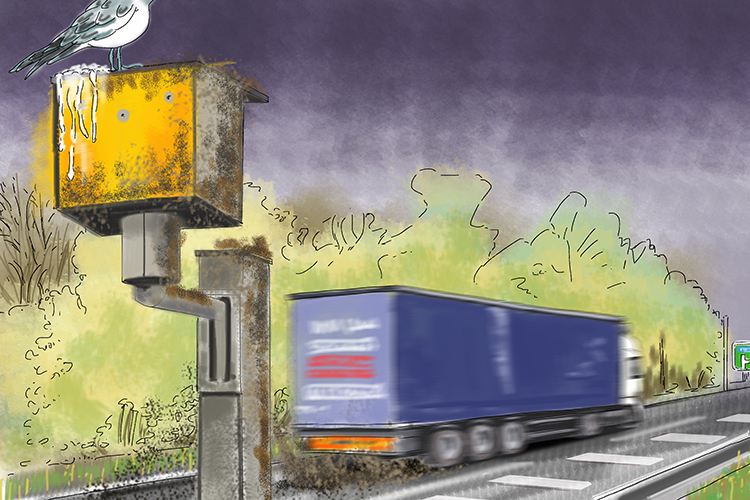# Physics formulas

Here are the physics equations you need. Yes, some exams provide them, but you may only have two hours. Knowing these equations off by heart may save you 10 minutes looking them up in an exam, ie 8% of your time, and the equation format may not be familiar. Make it easy on yourself and practise reeling off these formulas to get an A grade. After all, it’s not that difficult with our method.Save time.

## Speed

Speed is what we use to measure how fast something is moving. Speed is calculated using the formula below:Speed cameras get dirty (distance) over time.

NOTE:

The greater an object's speed the faster it is moving, and therefore the less time it will take to cover a certain distance.

Be careful: speed can be measured in several ways, for example miles per hour (mph), kilometres per hour (kph) and metres per second (m//s). Metres per second (m//s) is the standard unit for speed and is therefore the one you will most likely be asked for in an exam, but always read the question carefully to see how the answer needs to be presented.

Example 1

Ahmed drives 15\ km at an average speed of 60\ km//h. How long does the journey take?

speed=d/t

To answer this question you must first rearrange the formula to make time the subject. Rearranging a formula is easy so long as you remember that what you do to one side of an equation you do to the other. Therefore:

speed=(di\st\a\n\ce)/(time)

Multiply both sides by time to make distance the subject (the variable by itself on either the left or right side of the equation):

speed\times\time=(di\st\a\n\cetimescancel(time))/cancel(time)

now divide both sides by speed to make time the subject:

(cancel(speed)\times\time)/cancel(speed)=(di\st\a\n\ce)/(speed)

time=(di\st\a\n\ce)/(speed)

time=(15\ km)/(60\ kmph)

which is equal to 0.25 hours.

Now as we don’t measure time in decimals of an hour we need to convert this to minutes or seconds. An hour contains 60 minutes so:

0.25\ hours=0.25xx60\ mi\n\u\t\es=15\ mi\n\u\t\es

If we wanted the answer in seconds we need to convert 15\ mi\n\u\t\es into seconds. As a minutes contains 60 seconds 15\ mi\n\u\t\es=15xx60\ se\co\nds=900\ se\co\nds

Example 2

Penny cycles to work at 18km//h and it takes her 20 minutes. How far does she cycle to work?

speed=d/t

To answer this question you must first rearrange the formula to make distance the subject. Therefore:

speed=(di\st\a\n\ce)/(time)

Multiply both sides by time to make distance the subject,

speed\times\time=(di\st\a\n\cetimescancel(time))/cancel(time)

dis\t\a\n\ce=speed\times\time

The next thing we must do is make sure we are using compatible measurements for each parameter. The speed is given in km//h but the time is in minutes, so you must convert the speed to km per minute or the time to hours.

20\ mi\n\u\t\es=1/3hour

Therefore:

dis\t\a\n\ce=18times1/3=6\ km

Example 3

A car sets off on a journey at 7:43am and travels at an average speed of 50mph until 9:39pm. How far does the car travel to the nearest mile?

speed=d/t

To answer this question you must first rearrange the formula to make distance the subject. Therefore:

speed=(di\st\a\n\ce)/(time)

Multiply both sides by time to make distance the subject,

speed\times\time=(di\st\a\n\cetimescancel(time))/cancel(time)

dis\t\a\n\ce=speed\times\time

The next thing we must do is work out how long the car was travelling for. 7:43am to 9:39pm is 13hours and 56 minutes.

You could now either convert the whole journey time into minutes and the speed into miles per minute to calculate the distance, or work out how far the car would travel in 14 hours and then subtract how far it would travel in 4 minutes.

Distance after 14 hours =50xx14=700 miles

To work out how far the car travels in 4 minutes you must convert the speed from miles per hour to miles per minute, so as there are 60 minutes in an hour all you have to do is divide the speed by 60.

dis\t\a\n\ce=(50\ kmph)/60\times\ 4\ min=3\1/3\ mi\l\e\s

Therefore the total journey distance is:

700-3\ 1/3=696\ 2/3\ mi\l\e\s

To the nearest mile this is 697 miles